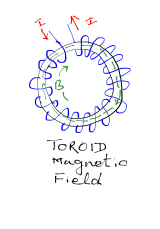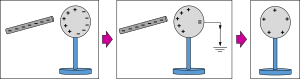## Pages

Showing posts with label class12-Physics. Show all posts
Showing posts with label class12-Physics. Show all posts

## Wednesday, 8 February 2017

### CBSE Class 12 - Marking Scheme of Physics Sample Question Paper (2016-17) (#cbsePapers)

CBSE Class 12  - Marking Scheme of Physics Sample Question Paper (2016-17)

See Class 12 Physics Sample Question Paper (2016-17)## Monday, 6 February 2017

### CBSE Class 12 - Physics Sample Question Paper (2016-17) (#cbsepapers)

CBSE Class 12  - Physics Sample Question Paper (2016-17)

See Class 12 Physics Marking Scheme SQP (2016-17)## Wednesday, 18 May 2016

### Class 12 - Physics - Chapter 4: Moving Charges and Magnetism (VSQA)

Moving Charges and Magnetism

### Very Short Q & A

Q1: Define Magnetic Field.

Answer: The space around a magnet within which its effect is experienced is called Magnetic Field (B).

Q2: What is the SI unit of magnetic field?

Answer: Tesla. Another unit is Newton Ampere-1 metre-1

Q3: What is a solenoid?

Answer: A solenoid is a circular coil of a large number of turns, such that the turns of solenoid run parallel to its length. It acts similar to a bar magnet.

Q4: What is Lorentz force?

Answer: The total force on a charge (q) moving with a velocity (v) in the presence of magnetic and electric field (B and F) respectively is called Lorentz force.

Q5: What is a cyclotron?

Answer: It is a machine to accelerate charged particles or ions to high energies

Q6: What is the operational definition of one ampere?## Sunday, 20 March 2016

### CBSE Class 12 - Physics - Electric Charges And Fields - Very Short Q and A

Electric Charges And Fields

### Very Short Q and ACharging By Induction credits:openclipart

Q1: Define frictional electricity.

Answer: It is the electricity developed on bodies when they rubbed against each other.

Q2: What is the smallest amount of charge?

Answer: Charge on an electron or proton is ± 1.62 × 10-19 C

Q3: State the two basic properties of electric charge?

Answer:  i. Electric charge is quantised.
ii. Electric charge is always conserved.

Q4: Define Dielectric constant.

Answer: The Dielectric constant of a medium is the ratio of the force between two charges placed at a certain distance apart in vacuum(or air) to the force between the same two charges at the same distance apart in that medium.

Q5: What is induction?

Answer: It is a process of charging a body without making physical contact.## Thursday, 28 January 2016

### CBSE Class 12 - Physics- Marking Scheme- Sample Question Paper (2016)

CBSE Class 12 - Physics- Marking Scheme- Sample Question Paper (2016)## Tuesday, 15 December 2015

### CBSE Class 12 - Physics - Sample Question Paper (2015)

CBSE Class 12 - Physics- Sample Question Paper (2015)## Current Electricity

NCERT Solution

Q1: The storage battery of a car has an emf of 12 V. If the internal resistance of the battery is 0.4Ω, what is the maximum current that can be drawn from the battery?

Answer: Emf of the battery, E = 12 V
Internal resistance of the battery, r = 0.4 Ω
Maximum current drawn from the battery = I

According to Ohm’s law,
E = Ir
I = E/r  = 12/0.4 = 30 A
Thus, the maximum current drawn from the given battery is 30 A.

Q2: A battery of emf 10 V and internal resistance 3 Ω is connected to a resistor. If the current in the circuit is 0.5 A, what is the resistance of the resistor? What is the terminal voltage of the battery when the circuit is closed?

Emf of the battery, E = 10 V
Internal resistance of the battery, r = 3 Ω
Current in the circuit, I = 0.5 A
Resistance of the resistor = R
Applying Ohm’s law,
I = E/(R + r)
R + r = E/I  = 10/0.5 = 20 Ω
∴ 20 - 3 = 17 Ω

Terminal voltage of the resistor = V
According to Ohm’s law,
V = IR = 0.5 × 17 = 8.5 V
∴ the resistance of the resistor is 17 Ω and the terminal voltage is 8.5 V.

Q3: a) Three resistors 1 Ω, 2 Ω, and 3 Ω are combined in series. What is the total resistance of the combination?
b) If the combination is connected to a battery of emf 12 V and negligible internal resistance, obtain the potential drop across each resistor.